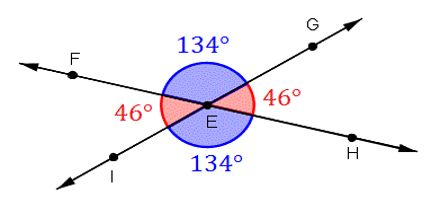Mathematic

# Lecture on Vertical AnglesPrinciple purpose of this lecture is to present on Vertical Angles. Vertical Angles is a pair of non-adjacent angles formed by the intersection of two straight lines. They are the angles that are opposite each other when two straight lines intersect. (Technically, these two lines need to be on the same plane). Vertical Angles are the angles opposite each other when two lines cross. They are always equal.